## Selina Concise Mathematics Class 10 ICSE Solutions Chapter 9 Matrices Ex 9C

These Solutions are part of Selina Concise Mathematics Class 10 ICSE Solutions. Here we have given Selina Concise Mathematics Class 10 ICSE Solutions Chapter 9 Matrices Ex 9C.

Other Exercises

Question 1.
Evaluate if possible :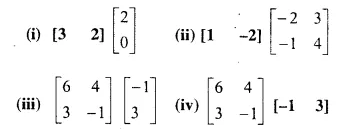If not possible, give a reason.
Solution: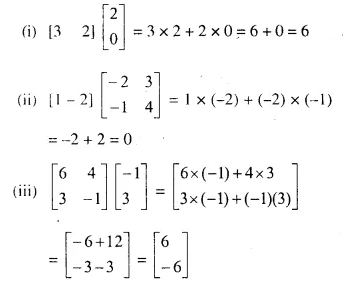(iv) It is not possible, because number of columns of the first matrix is not equal to number of rows of the second matrix.

Question 2.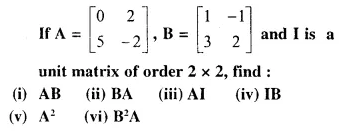Solution: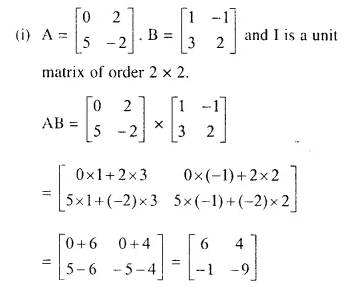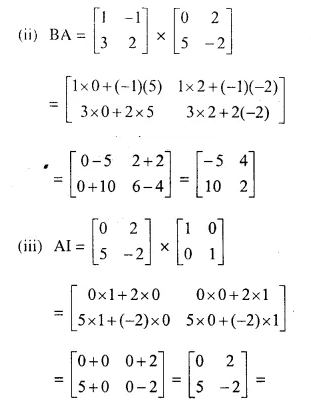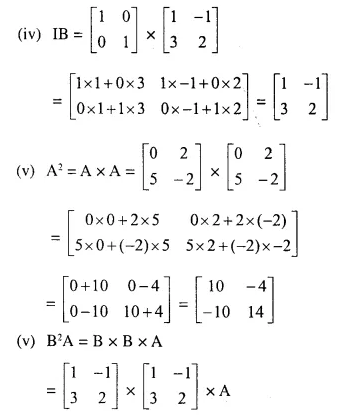Question 3.Solution: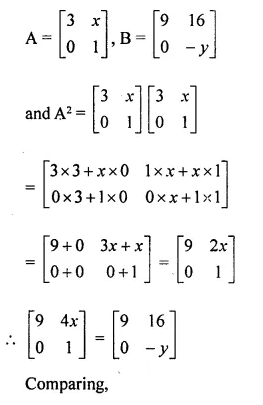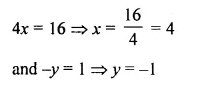Question 4.
Find x and y, if:Solution: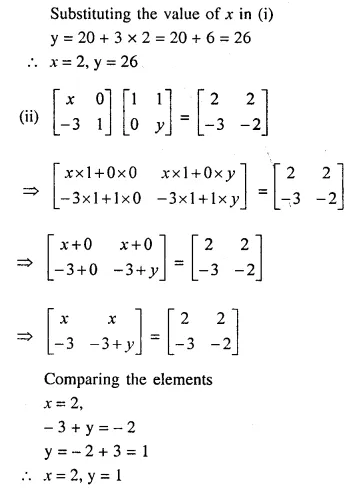Question 5.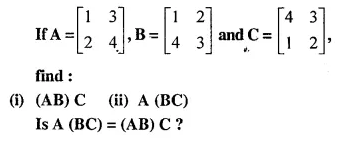Solution: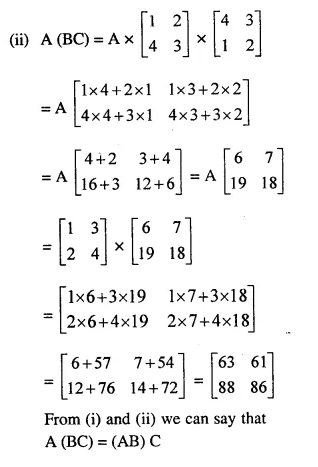Question 6.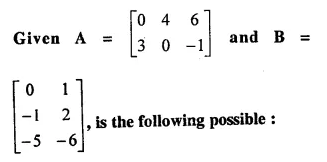(i) AB
(ii) BA
(iii) A²
Solution:Question 7.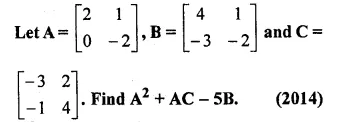Solution: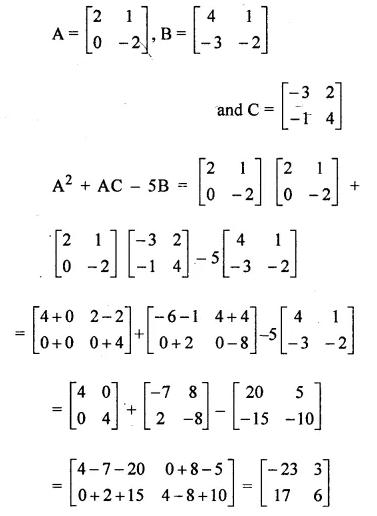Question 8.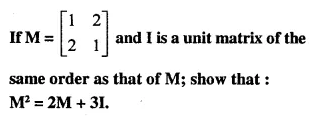Solution: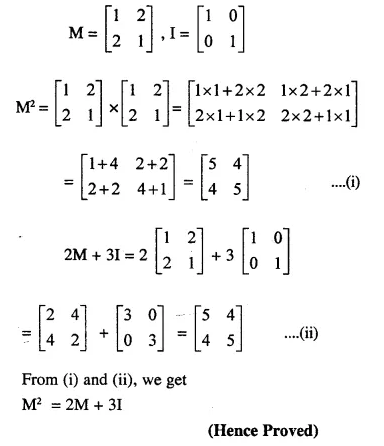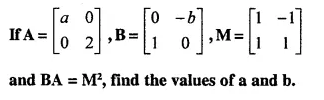Question 9.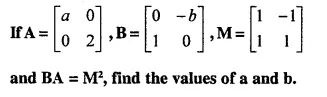Solution:Comparing the elements, we get:
-2b =-2
b = 1
a = 2

Question 10.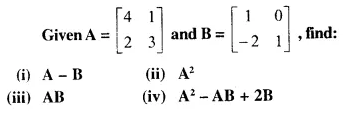Solution: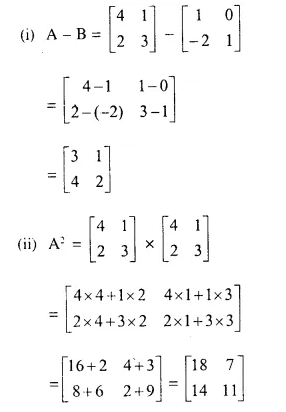Question 11.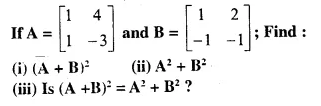Solution: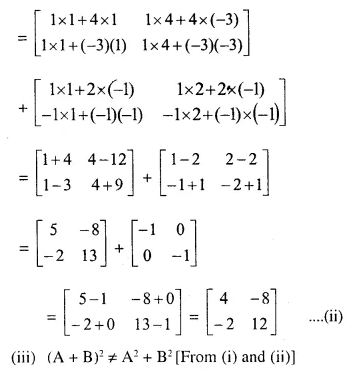Question 12.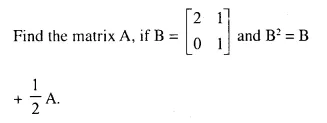Solution: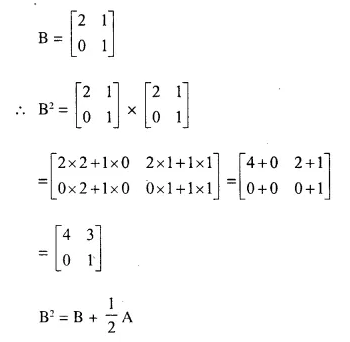Question 13.Solution:Question 14.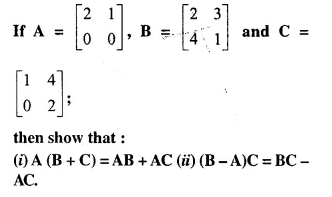Solution: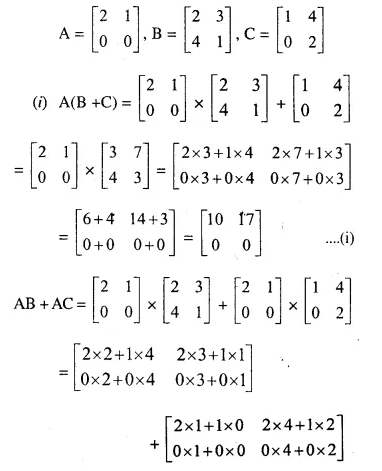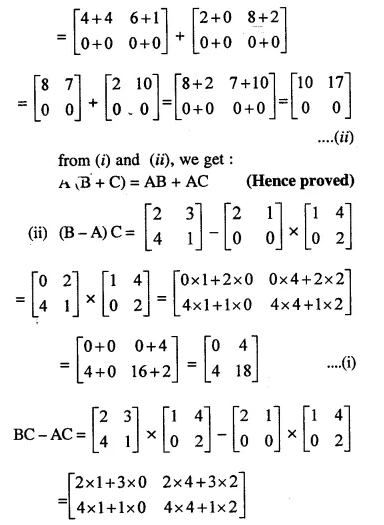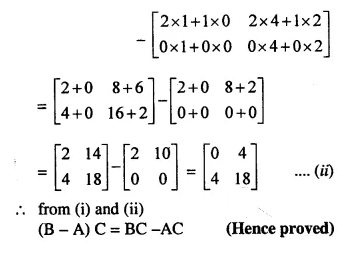Question 15.Simplify : A² + BC.
Solution: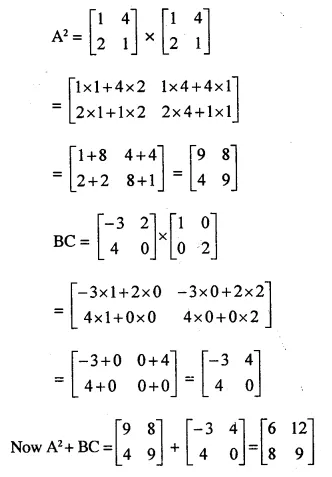Question 16.
Solve for x and y :Solution: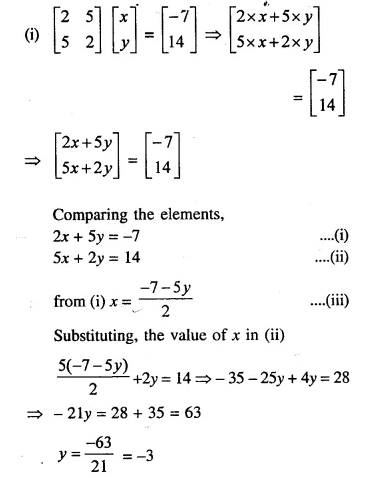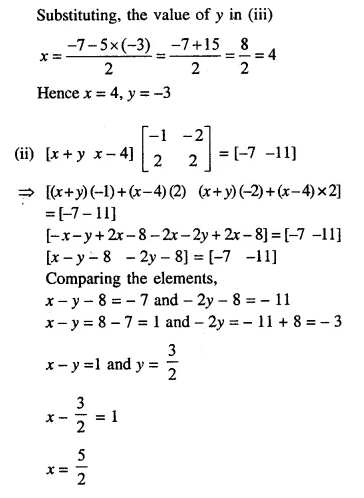Question 17.
In each case given below, find :
(a) the order of matrix M.
(b) the matrix M.Solution: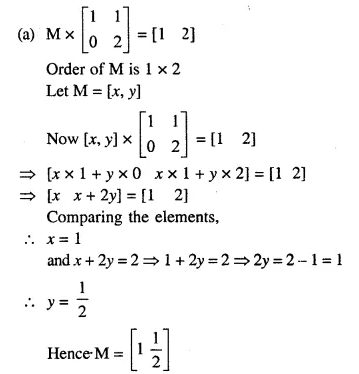Question 18.Solution:Question 19.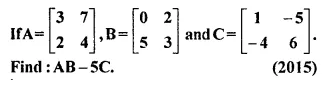Solution: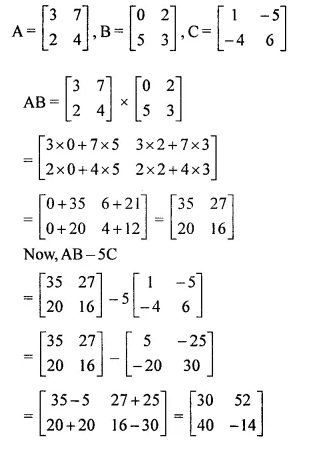Question 20.
If A and B are any two 2 x 2 matrices such that AB = BA = B and B is not a zero matrix, What can you say about the matrix A?
Solution:
AB = BA = B
But it is possible, when A = 0 or B = 0
But B is not a zero matrix (given)
A is a zero matrix or A is an identity matrix

Question 21.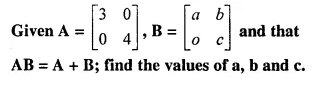Solution: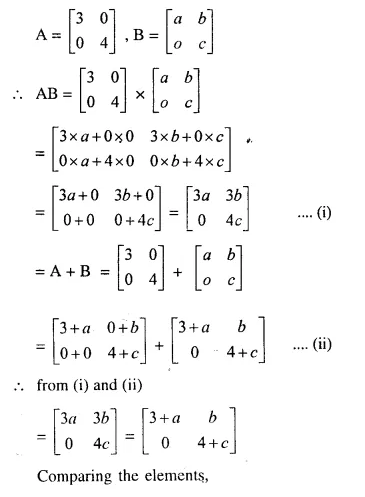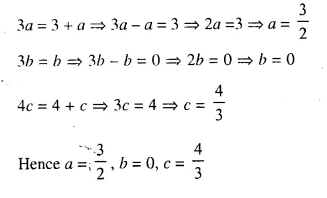Question 22.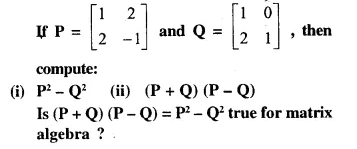Solution: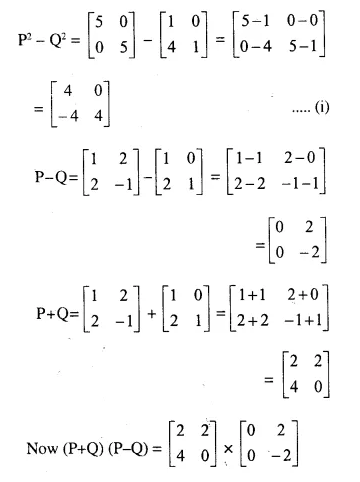Question 23.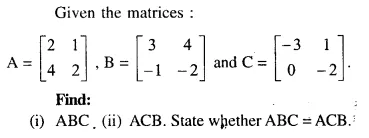Solution: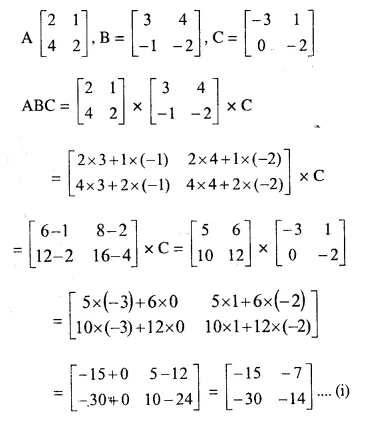Question 24.Solution: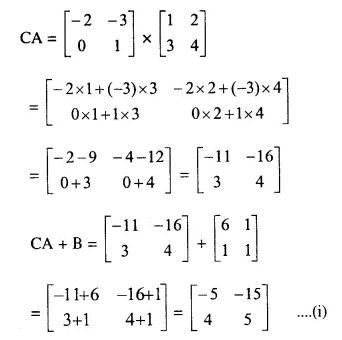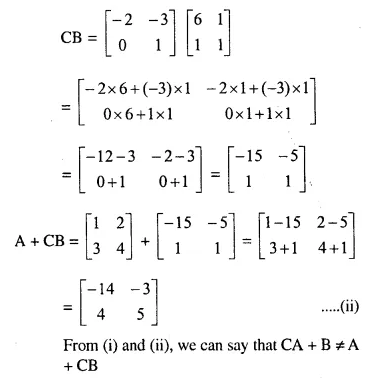Question 25.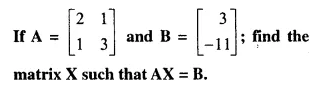Solution: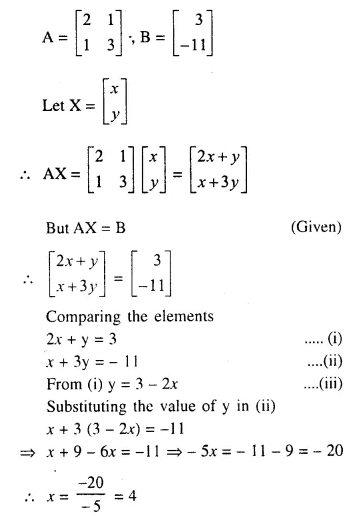Question 26.Solution: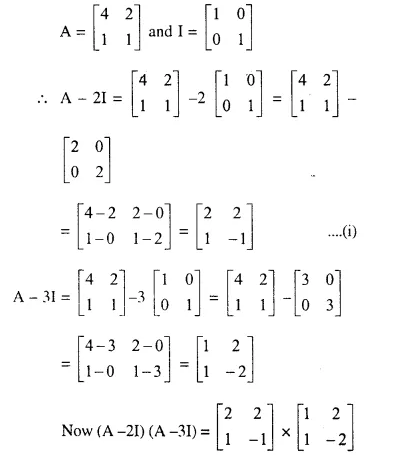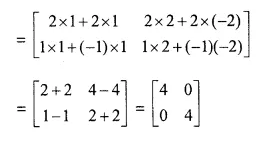Question 27.Solution: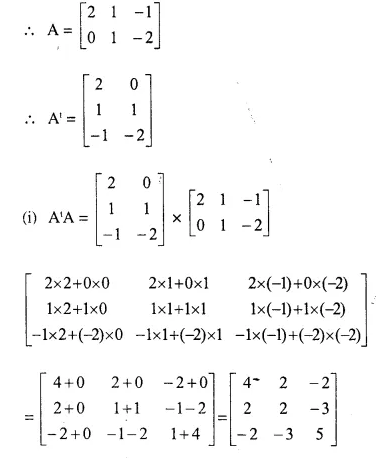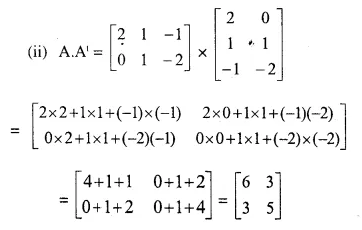Question 28.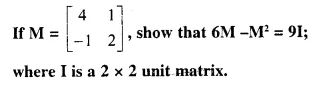Solution: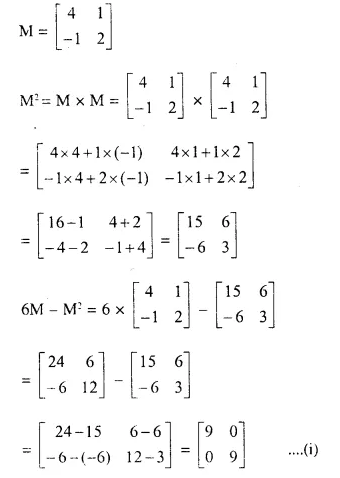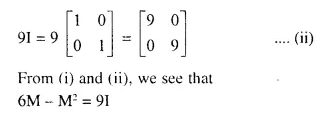Question 29.Solution: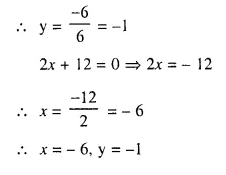Question 30.Solution: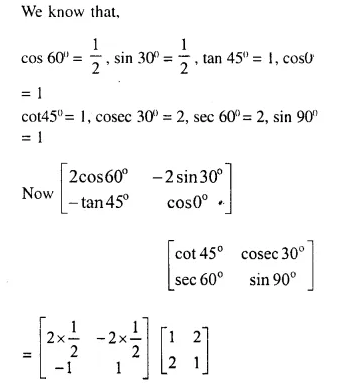Question 31.
State, with reason, whether the following are true or false. A, B and C are matrices of order 2 x 2.
(i) A + B = B + A
(ii) A – B = B – A
(iii) (B . C). A = B . (C . A)
(iv) (A + B) . C = A . C + B . C
(v) A . (B – C) = A . B – A . C
(vi) (A – B) . C = A . C – B . C
(vii) A² – B² = (A + B) (A – B)
(viii) (A – B)² = A² – 2 A . B + B²
Solution:
(i) True : Because addition of matrices is commutative.
(ii) False : Subtraction of matrices is not commutative.
(iii) True : Multiplication of matrices is associative.
(iv) True: Multiplication of matrices is distributive over addition.
(v) True : As given above in (iv)
(vi) True : As given above in (iv)
(vii) False : Laws of algebra for factorization and expansion are not applicable to matrices.
(viii) False, As given above in (vii)

Hope given Selina Concise Mathematics Class 10 ICSE Solutions Chapter 9 Matrices Ex 9C are helpful to complete your math homework.

If you have any doubts, please comment below. Learn Insta try to provide online math tutoring for you.